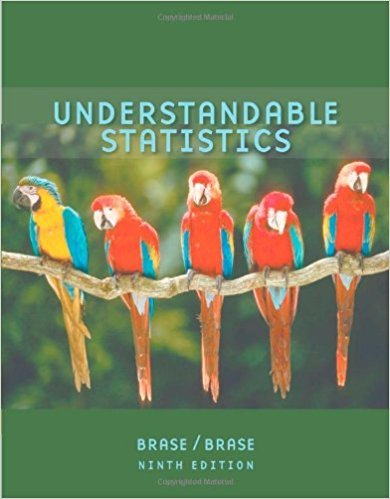×
×

# Solutions for Chapter 3: Organizing Data## Full solutions for Understandable Statistics | 9th Edition

ISBN: 9780618949922Solutions for Chapter 3: Organizing Data

Solutions for Chapter 3
4 5 0 346 Reviews
23
5
##### ISBN: 9780618949922

This textbook survival guide was created for the textbook: Understandable Statistics, edition: 9. Since 11 problems in chapter 3: Organizing Data have been answered, more than 33263 students have viewed full step-by-step solutions from this chapter. This expansive textbook survival guide covers the following chapters and their solutions. Chapter 3: Organizing Data includes 11 full step-by-step solutions. Understandable Statistics was written by and is associated to the ISBN: 9780618949922.

Key Statistics Terms and definitions covered in this textbook
• `-error (or `-risk)

In hypothesis testing, an error incurred by rejecting a null hypothesis when it is actually true (also called a type I error).

A formula used to determine the probability of the union of two (or more) events from the probabilities of the events and their intersection(s).

• Attribute

A qualitative characteristic of an item or unit, usually arising in quality control. For example, classifying production units as defective or nondefective results in attributes data.

• Binomial random variable

A discrete random variable that equals the number of successes in a ixed number of Bernoulli trials.

• Causal variable

When y fx = ( ) and y is considered to be caused by x, x is sometimes called a causal variable

• Center line

A horizontal line on a control chart at the value that estimates the mean of the statistic plotted on the chart. See Control chart.

• Coeficient of determination

See R 2 .

• Conditional probability

The probability of an event given that the random experiment produces an outcome in another event.

• Consistent estimator

An estimator that converges in probability to the true value of the estimated parameter as the sample size increases.

• Continuous random variable.

A random variable with an interval (either inite or ininite) of real numbers for its range.

• Contour plot

A two-dimensional graphic used for a bivariate probability density function that displays curves for which the probability density function is constant.

• Counting techniques

Formulas used to determine the number of elements in sample spaces and events.

• Crossed factors

Another name for factors that are arranged in a factorial experiment.

• Defects-per-unit control chart

See U chart

• Dependent variable

The response variable in regression or a designed experiment.

• Error propagation

An analysis of how the variance of the random variable that represents that output of a system depends on the variances of the inputs. A formula exists when the output is a linear function of the inputs and the formula is simpliied if the inputs are assumed to be independent.

• Expected value

The expected value of a random variable X is its long-term average or mean value. In the continuous case, the expected value of X is E X xf x dx ( ) = ?? ( ) ? ? where f ( ) x is the density function of the random variable X.

• First-order model

A model that contains only irstorder terms. For example, the irst-order response surface model in two variables is y xx = + ?? ? ? 0 11 2 2 + + . A irst-order model is also called a main effects model

• Generator

Effects in a fractional factorial experiment that are used to construct the experimental tests used in the experiment. The generators also deine the aliases.

• Hat matrix.

In multiple regression, the matrix H XXX X = ( ) ? ? -1 . This a projection matrix that maps the vector of observed response values into a vector of itted values by yˆ = = X X X X y Hy ( ) ? ? ?1 .

×